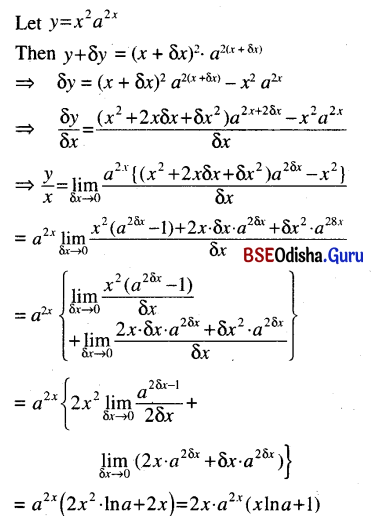# CHSE Odisha Class 12 Math Solutions Chapter 7 Continuity and Differentiability Ex 7(b)

Odisha State Board Elements of Mathematics Class 12 CHSE Odisha Solutions Chapter 7 Continuity and Differentiability Ex 7(b) Textbook Exercise Questions and Answers.

## CHSE Odisha Class 12 Math Solutions Chapter 7 Continuity and Differentiability Exercise 7(b)

Question 1.
Differentiate from definition
(i) e3x
Solution: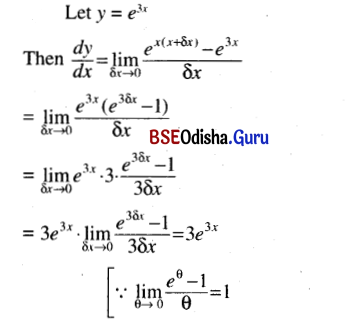(ii) 2x2
Solution: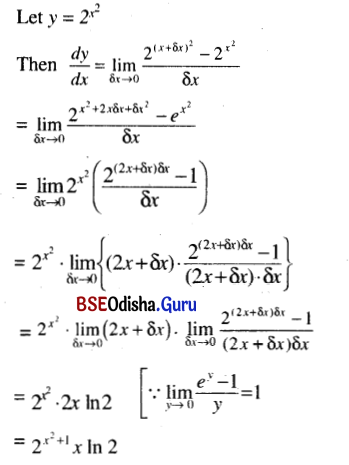(iii) In (3x + 1)
Solution: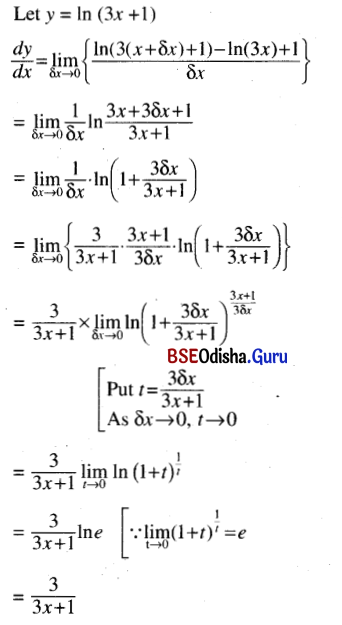(iv) logx5 (Hint : logx5 = $$\frac{\ln 5}{\ln x}$$)
Solution: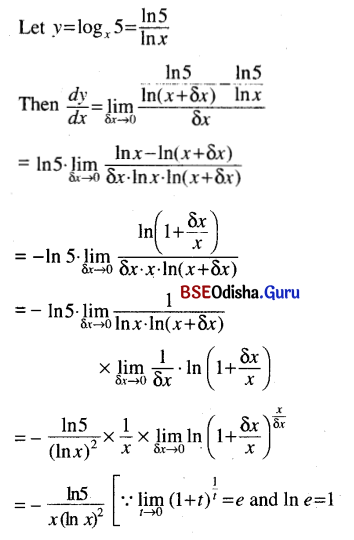(v) In sin x
Solution: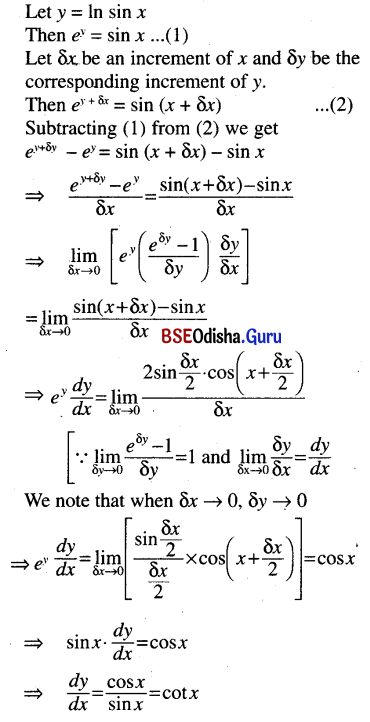(vi) x2 a2x
Solution: